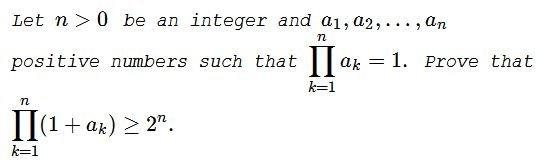# Two Products: Constraint and Inequality

### Problem### Proof 1

Since $\displaystyle\prod_{k=1}^na_k = 1,\,$

$\displaystyle\prod_{k=1}^n\left(1+\frac{1}{a_k}\right) = \prod_{k=1}^n(1+a_k)/\prod_{k=1}^na_k=\prod_{k=1}^n(1+a_k).$

Thus, if, say, $\displaystyle\prod_{k=1}^n(1+a_k)=A,\,$ then

\displaystyle\begin{align} A^2 &= \prod_{k=1}^n\left(1+\frac{1}{a_k}\right)\prod_{k=1}^n(1+a_k)\\ &=\prod_{k=1}^n\left(1+\frac{1}{a_k}\right)(1+a_k)\\ &=\prod_{k=1}^n\left(1+1+a_k+\frac{1}{a_k}\right)\\ &\ge \prod_{k=1}^n(1+1+2) = 4^n\\ &= 2^{2n}, \end{align}

implying that $A\ge 2^n,\,$ with equality only when $a_1=a_2=\ldots=a_n=1.$

### Proof 2

By the AM-GM inequality,

$\displaystyle\prod_{k=1}^n(1+a_k)\ge\prod_{k=1}^n2\sqrt{a_k}=2^n\sqrt{\prod_{k=1}^na_k}=2^n.$

### Proof 3

By Hölder's inequality,

$\displaystyle\prod_{k=1}^n(1+a_k)\ge\left(1+\sqrt[n]{\prod_{k=1}^na_k}\right)^n=2^n.$

### Proof 4

That's a proof by induction.

For $n=1\,$ there is nothing to prove. Let's check the case of $n=2.\,$ We have $a_1a_2=1\,$ and need to prove that $(1+a_1)(1+a_2)\ge 4.\,$ But

$(1+a_1)(1+a_2)=1+a_1+a_2+a_1a_2=2+(a_1+a_2)\ge 2+2\sqrt{a_1a_2}=4.$

Assume the truth of the statement for some $n\ge 2\,$ and consider $n+1\,$ positive numbers such that $\displaystyle\prod_{k=1}^{n+1}a_k=1.\,$ One consequence of that condition is that there are bound to be two numbers, say, $a_{n}\le 1\,$ and $a_{n+1}\ge 1.\,$ By the inductive assumption, $\displaystyle\prod_{k=1}^{n-1}(1+a_k)(1+a_{n}a_{n+1})\ge 2^{n}.\,$ We thus have

\displaystyle\begin{align} &0 \ge (1-a_{n-1})(1-a_n),\\ &a_{n-1}+a_n\ge 1 +a_{n-1}a_n,\\ &\frac{1}{2}(1+a_{n-1})(1+a_{n})\ge 1 +a_{n-1}a_n,\\ &\prod_{k=1}^{n-1}(1+a_k)\cdot \frac{1}{2}(1+a_{n-1})(1+a_{n})\ge\prod_{k=1}^{n-1}(1+a_k)(1 +a_{n-1}a_n)\ge 2^n,\\ &\prod_{k=1}^{n+1}(1+a_k)\ge 2^{n+1}. \end{align}

### Modification

Let $n\gt 0\,$ be an integer and $a_1, a_2, \ldots, a_n\,$ positive numbers such that $\displaystyle\prod_{k=1}^na_k = 1.\,$ Prove that $\displaystyle\prod_{k=1}^n(1+a_k+a_{k+1})\ge 3^n,$

where $a_{n+1}=a_1.$

### Acknoweledgment

The problem came from an old Russian book (Ye. Vachovsky, A. Ryvkin, Problems in Elementary Mathematics of Increased Level of Difficulty, Nauka, 1971).

Solution 1 is from the book; Solutions 2 and 3 are by Leo Giugiuc.### Inequalities with the Product of Variables as a Constraint